• 蚁群算法求解旅行商问题 蚁群算法最初是通过对蚂蚁群落的观察，受蚁群行为特征启发而得出的。蚂蚁是一种群居昆虫，在觅食、清理巢穴等活动中，彼此依赖、相互协作共同完成特定的任务。就个体来讲，单个蚂蚁的智力和...
• 分 数_ 任课教师签字_ 华北电力大学研究生结课作业 学 年 学 期20 10 学年第二学期 课 程 名 称人工智能与知识工程 学 生 姓 名张华壮 学 号2 10222 1007 题 目蚁群算法概述 提 交 时 间20 11/4/ 13 1 蚁群算法概述 ...
• 配送网络规划蚁群算法 蚁群算法的研究现状和应用及蚂蚁智能体的硬件实现.pdf 蚁群算法求解问题时易产生的误区及对策.pdf 蚁群算法求解连续空间优化问题的一种方法.pdf 蚁群算法在啤酒发酵控制优化中的应用.pdf 用...
• 一些经典蚁群算法，从基本蚁群算法、最大最小蚂蚁系统、简化最大最小蚂蚁系统、基于最近邻最大最小蚂蚁系统、蚁群系统，排序蚂蚁系统、精英蚂蚁系统到自适应蚁群算法，用于TSP问题求解。
• matlab解决TSP问题蚁群算法-蚁群算法解决TSP问题.doc 在求解TSP问题中有比较好的应用，大家可以看一下！！matlab
• 采用蚁群算法解决TSP旅行商问题。先用dijkstra算法生成初始次优路径，再用蚁群算法搜索全局最优路径。
• Tsp蚁群算法仿真情况，可以通过学习，更好的了解蚁群算法
• 蚁群算法解TSP问题MATLAB;;蚁群算法解TSP问题MATLAB;蚁群算法解TSP问题MATLAB;蚁群算法解TSP问题MATLAB;蚁群算法解TSP问题MATLAB;蚁群算法解TSP问题MATLABTSP matlab
• 根据蚁群算法解决三维路径规划的问题，得到三维的路径图
• 1. 蚁群算法简介 蚁群算法（Ant Clony Optimization， ACO）是一种群智能算法，它是由一群无智能或有轻微智能的个体（Agent）通过相互协作而表现出智能行为，从而为求
原文地址为：
蚁群算法java实现以及TSP问题蚁群算法求解

1. 蚁群算法简介
蚁群算法（Ant Clony Optimization， ACO）是一种群智能算法，它是由一群无智能或有轻微智能的个体（Agent）通过相互协作而表现出智能行为，从而为求解复杂问题提供了一个新的可能性。蚁群算法最早是由意大利学者Colorni A., Dorigo M. 等于1991年提出。经过20多年的发展，蚁群算法在理论以及应用研究上已经得到巨大的进步。
蚁群算法是一种仿生学算法，是由自然界中蚂蚁觅食的行为而启发的。在自然界中，蚂蚁觅食过程中，蚁群总能够按照寻找到一条从蚁巢和食物源的最优路径。图（1）显示了这样一个觅食的过程。图（1）蚂蚁觅食

在图1（a）中，有一群蚂蚁，假如A是蚁巢，E是食物源（反之亦然）。这群蚂蚁将沿着蚁巢和食物源之间的直线路径行驶。假如在A和E之间突然出现了一个障碍物（图1（b）），那么，在B点（或D点）的蚂蚁将要做出决策，到底是向左行驶还是向右行驶？由于一开始路上没有前面蚂蚁留下的信息素（pheromone），蚂蚁朝着两个方向行进的概率是相等的。但是当有蚂蚁走过时，它将会在它行进的路上释放出信息素，并且这种信息素会议一定的速率散发掉。信息素是蚂蚁之间交流的工具之一。它后面的蚂蚁通过路上信息素的浓度，做出决策，往左还是往右。很明显，沿着短边的的路径上信息素将会越来越浓（图1（c）），从而吸引了越来越多的蚂蚁沿着这条路径行驶。
2. TSP问题描述
蚁群算法最早用来求解TSP问题，并且表现出了很大的优越性，因为它分布式特性，鲁棒性强并且容易与其它算法结合，但是同时也存在这收敛速度慢，容易陷入局部最优（local optimal）等缺点。
TSP问题（Travel Salesperson Problem，即旅行商问题或者称为中国邮递员问题），是一种，是一种NP-hard问题，此类问题用一般的算法是很大得到最优解的，所以一般需要借助一些启发式算法求解，例如遗传算法（GA），蚁群算法（ACO），微粒群算法（PSO）等等。
TSP问题可以分为两类，一类是对称TSP问题（Symmetric TSP），另一类是非对称问题（Asymmetric TSP）。所有的TSP问题都可以用一个图（Graph）来描述：
令

$V=\{c_1,c_2,\ldots,c_i,\ldots,c_n\},i=1,2,\ldots,n$是所有城市的集合. $c_i$表示第i个城市， $n$为城市的数目；

$E=\{(r,s):r,s \in V\}$是所有城市之间连接的集合；

$C=\{c_{rs}:r,s \in V\}$是所有城市之间连接的成本度量（一般为城市之间的距离）；

如果$c_{rs} = c_{sr}$, 那么该TSP问题为对称的，否则为非对称的。

一个TSP问题可以表达为：
求解遍历图$G=(V,E,C)$，所有的节点一次并且回到起始节点，使得连接这些节点的路径成本最低。
3. 蚁群算法原理
假如蚁群中所有蚂蚁的数量为m，所有城市之间的信息素用矩阵pheromone表示，最短路径为bestLength，最佳路径为bestTour。每只蚂蚁都有自己的内存，内存中用一个禁忌表（Tabu）来存储该蚂蚁已经访问过的城市，表示其在以后的搜索中将不能访问这些城市；还有用另外一个允许访问的城市表（Allowed）来存储它还可以访问的城市；另外还用一个矩阵（Delta）来存储它在一个循环（或者迭代）中给所经过的路径释放的信息素；还有另外一些数据，例如一些控制参数$(\alpha，\beta，\rho，Q)$，该蚂蚁行走玩全程的总成本或距离（tourLength），等等。假定算法总共运行MAX_GEN次，运行时间为t。
蚁群算法计算过程如下：
（1）初始化

设t=0，初始化bestLength为一个非常大的数（正无穷），bestTour为空。初始化所有的蚂蚁的Delt矩阵所有元素初始化为0，Tabu表清空，Allowed表中加入所有的城市节点。随机选择它们的起始位置（也可以人工指定）。在Tabu中加入起始节点，Allowed中去掉该起始节点。

（2）为每只蚂蚁选择下一个节点。
为每只蚂蚁选择下一个节点，该节点只能从Allowed中以某种概率（公式1）搜索到，每搜到一个，就将该节点加入到Tabu中，并且从Allowed中删除该节点。该过程重复n-1次，直到所有的城市都遍历过一次。遍历完所有节点后，将起始节点加入到Tabu中。此时Tabu表元素数量为n+1（n为城市数量），Allowed元素数量为0。接下来按照（公式2）计算每个蚂蚁的Delta矩阵值。最后计算最佳路径，比较每个蚂蚁的路径成本，然后和bestLength比较，若它的路径成本比bestLength小，则将该值赋予bestLength，并且将其Tabu赋予BestTour。（公式1）

（公式2）
其中$p_{ij}^{(t)}$表示选择城市j的概率，$k$表示第$k$个蚂蚁，$\tau_{ij}^{(t)}$表示城市$i,j$在第$t$时刻的信息素浓度，$\eta_{ij}$表示从城市i到城市j的可见度，
$\eta_{ij} = \frac 1 {d_{ij}}$，$d_{ij}$表示城市$i,j$之间的成本（或距离）。由此可见$d_{ij}$越小，$\eta_{ij}$越大，也就是从城市$i$到$j$的可见性就越大。$\Delta \tau_{ij}^k$表示蚂蚁$k$在城市$i$与$j$之间留下的信息素。
$L_k$表示蚂蚁$k$经过一个循环（或迭代）锁经过路径的总成本（或距离），即tourLength.$\alpha, \beta, Q$ 均为控制参数。

（3）更新信息素矩阵

令$t=t+n$t，按照（公式3）更新信息素矩阵phermone。
$\tau_{ij}(t+n) = \rho \cdot \tau_{ij}(t) + \Delta \tau_{ij}$
（公式3）
$\tau_{ij}(t+n)$为$t+n$时刻城市$i$与$j$之间的信息素浓度。$\rho$为控制参数，$Delta_ij$为城市$i$与$j$之间信息素经过一个迭代后的增量。并且有
$\Delta \tau_{ij} = \sum_{k=1}^m \Delta \tau_{ij}^k$
（公式4）
其中$\Delta \tau_{ij}^k$由公式计算得到。

（4）检查终止条件

如果达到最大代数MAX_GEN，算法终止，转到第（5）步；否则，重新初始化所有的蚂蚁的Delt矩阵所有元素初始化为0，Tabu表清空，Allowed表中加入所有的城市节点。随机选择它们的起始位置（也可以人工指定）。在Tabu中加入起始节点，Allowed中去掉该起始节点，重复执行（2），（3）,(4)步。

（5）输出最优值
4. Java实现
在该java实现中我们选择使用tsplib上的数据att48，这是一个对称tsp问题，城市规模为48，其最优值为10628.其距离计算方法如图（2）所示：图（2）att48距离计算方法
实现中，使用了两个java类，一个Ant类，一个ACO类。
具体实现代码如下（此代码借鉴了蚁群优化算法的JAVA实现）：
Ant类：
1: import java.util.Random;
2: import java.util.Vector;
3:
4: /**
5:  *
6:  * @author BIAO YU
7:  *
8:  */
9: public class Ant implements Cloneable {
10:
11:   private Vector<Integer> tabu; //禁忌表
12:   private Vector<Integer> allowedCities; //允许搜索的城市
13:   private float[][] delta; //信息数变化矩阵
14:   private int[][] distance; //距离矩阵
15:
16:   private float alpha;
17:   private float beta;
18:
19:   private int tourLength; //路径长度
20:   private int cityNum; //城市数量
21:
22:   private int firstCity; //起始城市
23:   private int currentCity; //当前城市
24:
25:   public Ant(){
26:     cityNum = 30;
27:     tourLength = 0;
28:
29:   }
30:
31:   /**
32:    * Constructor of Ant
33:    * @param num 蚂蚁数量
34:    */
35:   public Ant(int num){
36:     cityNum = num;
37:     tourLength = 0;
38:
39:   }
40:
41:   /**
42:    * 初始化蚂蚁，随机选择起始位置
43:    * @param distance 距离矩阵
44:    * @param a alpha
45:    * @param b beta
46:    */
47:   public void init(int[][] distance, float a, float b){
48:     alpha = a;
49:     beta = b;
50:     allowedCities = new Vector<Integer>();
51:     tabu = new Vector<Integer>();
52:     this.distance = distance;
53:     delta = new float[cityNum][cityNum];
54:     for (int i = 0; i < cityNum; i++) {
55:       Integer integer = new Integer(i);
57:       for (int j = 0; j < cityNum; j++) {
58:         delta[i][j] = 0.f;
59:       }
60:     }
61:
62:     Random random = new Random(System.currentTimeMillis());
63:     firstCity = random.nextInt(cityNum);
64:     for (Integer i:allowedCities) {
65:       if (i.intValue() == firstCity) {
66:         allowedCities.remove(i);
67:         break;
68:       }
69:     }
70:
72:     currentCity = firstCity;
73:   }
74:
75:   /**
76:    * 选择下一个城市
77:    * @param pheromone 信息素矩阵
78:    */
79:   public void selectNextCity(float[][] pheromone){
80:     float[] p = new float[cityNum];
81:     float sum = 0.0f;
82:     //计算分母部分
83:     for (Integer i:allowedCities) {
84:       sum += Math.pow(pheromone[currentCity][i.intValue()], alpha)*Math.pow(1.0/distance[currentCity][i.intValue()], beta);
85:     }
86:     //计算概率矩阵
87:     for (int i = 0; i < cityNum; i++) {
88:       boolean flag = false;
89:       for (Integer j:allowedCities) {
90:
91:         if (i == j.intValue()) {
92:           p[i] = (float) (Math.pow(pheromone[currentCity][i], alpha)*Math.pow(1.0/distance[currentCity][i], beta))/sum;
93:           flag = true;
94:           break;
95:         }
96:       }
97:
98:       if (flag == false) {
99:         p[i] = 0.f;
100:       }
101:     }
102:
103:     //轮盘赌选择下一个城市
104:     Random random = new Random(System.currentTimeMillis());
105:     float sleectP = random.nextFloat();
106:     int selectCity = 0;
107:     float sum1 = 0.f;
108:     for (int i = 0; i < cityNum; i++) {
109:       sum1 += p[i];
110:       if (sum1 >= sleectP) {
111:         selectCity = i;
112:         break;
113:       }
114:     }
115:
116:     //从允许选择的城市中去除select city
117:     for (Integer i:allowedCities) {
118:       if (i.intValue() == selectCity) {
119:         allowedCities.remove(i);
120:         break;
121:       }
122:     }
123:     //在禁忌表中添加select city
125:     //将当前城市改为选择的城市
126:     currentCity = selectCity;
127:
128:   }
129:
130:   /**
131:    * 计算路径长度
132:    * @return 路径长度
133:    */
134:   private int calculateTourLength(){
135:     int len = 0;
136:     for (int i = 0; i < cityNum; i++) {
137:       len += distance[this.tabu.get(i).intValue()][this.tabu.get(i+1).intValue()];
138:     }
139:     return len;
140:   }
141:
142:
143:
144:   public Vector<Integer> getAllowedCities() {
145:     return allowedCities;
146:   }
147:
148:   public void setAllowedCities(Vector<Integer> allowedCities) {
149:     this.allowedCities = allowedCities;
150:   }
151:
152:   public int getTourLength() {
153:     tourLength = calculateTourLength();
155:   }
156:   public void setTourLength(int tourLength) {
157:     this.tourLength = tourLength;
158:   }
159:   public int getCityNum() {
160:     return cityNum;
161:   }
162:   public void setCityNum(int cityNum) {
163:     this.cityNum = cityNum;
164:   }
165:
166:   public Vector<Integer> getTabu() {
167:     return tabu;
168:   }
169:
170:   public void setTabu(Vector<Integer> tabu) {
171:     this.tabu = tabu;
172:   }
173:
174:   public float[][] getDelta() {
175:     return delta;
176:   }
177:
178:   public void setDelta(float[][] delta) {
179:     this.delta = delta;
180:   }
181:
182:   public int getFirstCity() {
183:     return firstCity;
184:   }
185:
186:   public void setFirstCity(int firstCity) {
187:     this.firstCity = firstCity;
188:   }
189:
190: }
191:
ACO类：
2: import java.io.FileInputStream;
3: import java.io.IOException;
5:
6: /**
7:  *
8:  * @author BIAO YU
9:  *
10:  *
11:  */
12: public class ACO {
13:
14:   private Ant[] ants; //蚂蚁
15:   private int antNum; //蚂蚁数量
16:   private int cityNum; //城市数量
17:   private int MAX_GEN; //运行代数
18:   private float[][] pheromone; //信息素矩阵
19:   private int[][] distance; //距离矩阵
20:   private int bestLength; //最佳长度
21:   private int[] bestTour; //最佳路径
22:
23:   //三个参数
24:   private float alpha;
25:   private float beta;
26:   private float rho;
27:
28:
29:   public ACO(){
30:
31:   }
32:   /** constructor of ACO
33:    * @param n 城市数量
34:    * @param m 蚂蚁数量
35:    * @param g 运行代数
36:    * @param a alpha
37:    * @param b beta
38:    * @param r rho
39:    *
40:   **/
41:   public ACO(int n, int m, int g, float a, float b, float r) {
42:     cityNum = n;
43:     antNum = m;
44:     ants = new Ant[antNum];
45:     MAX_GEN = g;
46:     alpha = a;
47:     beta = b;
48:     rho = r;
49:
50:   }
51:
52:   @SuppressWarnings("resource")
53:   /**
54:    * 初始化ACO算法类
55:    * @param filename 数据文件名，该文件存储所有城市节点坐标数据
56:    * @throws IOException
57:    */
58:   private void init(String filename) throws IOException{
59:     //读取数据
60:         int[] x;
61:         int[] y;
62:         String strbuff;
64:
65:         distance = new int[cityNum][cityNum];
66:         x = new int[cityNum];
67:         y = new int[cityNum];
68:         for (int i = 0; i < cityNum; i++) {
70:             String[] strcol = strbuff.split("");
71:             x[i] = Integer.valueOf(strcol);
72:             y[i] = Integer.valueOf(strcol);
73:         }
74:         //计算距离矩阵 ，针对具体问题，距离计算方法也不一样，此处用的是att48作为案例，它有48个城市，距离计算方法为伪欧氏距离，最优值为10628
75:         for (int i = 0; i < cityNum - 1; i++) {
76:             distance[i][i] = 0;  //对角线为0
77:             for (int j = i + 1; j < cityNum; j++) {
78:               double rij = Math.sqrt(((x[i] - x[j]) * (x[i] - x[j])+ (y[i] - y[j]) * (y[i] - y[j]))/10.0);
79:               int tij = (int) Math.round(rij);
80:               if (tij < rij) {
81:                 distance[i][j] = tij + 1;
82:                     distance[j][i] = distance[i][j];
83:         }else {
84:           distance[i][j] = tij;
85:                     distance[j][i] = distance[i][j];
86:         }
87:             }
88:         }
89:         distance[cityNum - 1][cityNum - 1] = 0;
90:
91:         //初始化信息素矩阵
92:         pheromone=new float[cityNum][cityNum];
93:         for(int i=0;i<cityNum;i++)
94:         {
95:             for(int j=0;j<cityNum;j++){
96:                 pheromone[i][j]=0.1f;  //初始化为0.1
97:             }
98:         }
99:         bestLength=Integer.MAX_VALUE;
100:         bestTour=new int[cityNum+1];
101:         //随机放置蚂蚁
102:         for(int i=0;i<antNum;i++){
103:             ants[i]=new Ant(cityNum);
104:             ants[i].init(distance, alpha, beta);
105:         }
106:   }
107:
108:   public void solve(){
109:
110:     for (int g = 0; g < MAX_GEN; g++) {
111:       for (int i = 0; i < antNum; i++) {
112:         for (int j = 1; j < cityNum; j++) {
113:           ants[i].selectNextCity(pheromone);
114:         }
116:         if (ants[i].getTourLength() < bestLength) {
117:           bestLength = ants[i].getTourLength();
118:           for (int k = 0; k < cityNum + 1; k++) {
119:             bestTour[k] = ants[i].getTabu().get(k).intValue();
120:           }
121:         }
122:         for (int j = 0; j < cityNum; j++) {
123:           ants[i].getDelta()[ants[i].getTabu().get(j).intValue()][ants[i].getTabu().get(j+1).intValue()] = (float) (1./ants[i].getTourLength());
124:           ants[i].getDelta()[ants[i].getTabu().get(j+1).intValue()][ants[i].getTabu().get(j).intValue()] = (float) (1./ants[i].getTourLength());
125:         }
126:       }
127:
128:       //更新信息素
129:       updatePheromone();
130:
131:        //重新初始化蚂蚁
132:           for(int i=0;i<antNum;i++){
133:
134:               ants[i].init(distance, alpha, beta);
135:           }
136:     }
137:
138:     //打印最佳结果
139:     printOptimal();
140:   }
141:
142:   //更新信息素
143:   private void updatePheromone(){
144:     //信息素挥发
145:         for(int i=0;i<cityNum;i++)
146:             for(int j=0;j<cityNum;j++)
147:                 pheromone[i][j]=pheromone[i][j]*(1-rho);
148:         //信息素更新
149:         for(int i=0;i<cityNum;i++){
150:             for(int j=0;j<cityNum;j++){
151:                 for (int k = 0; k < antNum; k++) {
152:           pheromone[i][j] += ants[k].getDelta()[i][j];
153:         }
154:             }
155:         }
156:   }
157:
158:   private void printOptimal(){
159:     System.out.println("The optimal length is: " + bestLength);
160:     System.out.println("The optimal tour is: ");
161:     for (int i = 0; i < cityNum + 1; i++) {
162:       System.out.println(bestTour[i]);
163:     }
164:   }
165:
166:   public Ant[] getAnts() {
167:     return ants;
168:   }
169:
170:   public void setAnts(Ant[] ants) {
171:     this.ants = ants;
172:   }
173:
174:   public int getAntNum() {
175:     return antNum;
176:   }
177:
178:   public void setAntNum(int m) {
179:     this.antNum = m;
180:   }
181:
182:   public int getCityNum() {
183:     return cityNum;
184:   }
185:
186:   public void setCityNum(int cityNum) {
187:     this.cityNum = cityNum;
188:   }
189:
190:   public int getMAX_GEN() {
191:     return MAX_GEN;
192:   }
193:
194:   public void setMAX_GEN(int mAX_GEN) {
195:     MAX_GEN = mAX_GEN;
196:   }
197:
198:   public float[][] getPheromone() {
199:     return pheromone;
200:   }
201:
202:   public void setPheromone(float[][] pheromone) {
203:     this.pheromone = pheromone;
204:   }
205:
206:   public int[][] getDistance() {
207:     return distance;
208:   }
209:
210:   public void setDistance(int[][] distance) {
211:     this.distance = distance;
212:   }
213:
214:   public int getBestLength() {
215:     return bestLength;
216:   }
217:
218:   public void setBestLength(int bestLength) {
219:     this.bestLength = bestLength;
220:   }
221:
222:   public int[] getBestTour() {
223:     return bestTour;
224:   }
225:
226:   public void setBestTour(int[] bestTour) {
227:     this.bestTour = bestTour;
228:   }
229:
230:   public float getAlpha() {
231:     return alpha;
232:   }
233:
234:   public void setAlpha(float alpha) {
235:     this.alpha = alpha;
236:   }
237:
238:   public float getBeta() {
239:     return beta;
240:   }
241:
242:   public void setBeta(float beta) {
243:     this.beta = beta;
244:   }
245:
246:   public float getRho() {
247:     return rho;
248:   }
249:
250:   public void setRho(float rho) {
251:     this.rho = rho;
252:   }
253:
254:
255:   /**
256:    * @param args
257:    * @throws IOException
258:    */
259:   public static void main(String[] args) throws IOException {
260:     ACO aco = new ACO(48, 100, 1000, 1.f, 5.f, 0.5f);
261:     aco.init("c://data.txt");
262:     aco.solve();
263:   }
264:
265: }
266:
5. 总结
蚁群算法和其它的启发式算法一样，在很多场合都得到了应用，并且取得了很好的结果。但是同样存在着很多的缺点，例如收敛速度慢，容易陷入局部最优，等等。对于这些问题，还需要进一步的研究和探索，另外蚁群算法的数学机理至今还没有得到科学的解释，这也是当前研究的热点和急需解决的问题之一。注：TSP数据文件以及两篇早期的关于蚁群算法的文章包含在附件中，请点击此处下载附件。
转载请注明本文地址：
蚁群算法java实现以及TSP问题蚁群算法求解

展开全文算法 java
• 蚁群算法TSP问题的matlab源代码；蚁群算法TSP问题的matlab源代码
• 使用蚁群算法求解TSP旅行商问题，精华蚂蚁，最大最小蚂蚁系统，基于最近邻最大最小蚂蚁系统，排序蚂蚁系统-RAS、自适应蚁群算法-自适应挥发系数
• 飞行器的航迹规划问题-蚁群算法和多目标粒子群算法的赛题应用，学习算法的最好应用案例，带讲解的算法。
• 蚁群算法求解TSP 问题源码，运用经典的蚁群算法，方便解读学习
• 基于蚁群算法求解tsp问题的论文，有助于快速了解蚁群算法的研究现状
• 该代码是蚁群算法解决TSP问题，简单易懂。通过一系列function函数实现最优路径的优化
• 使用 蚁群算法求解tsp 问题 运行程序时选择后缀为tsp的文件加载
• 本代码用matlab写的蚁群算法解决TSP问题，详细说明了蚁群算法来解决TSp问题的过程。
• 解决TSP问题的蚂蚁群算法，具有详细的代码解释，方便初学者学习
• ## 蚁群算法

万次阅读 多人点赞 2018-06-27 21:49:51
蚁群算法能够有效的解决著名的旅行商问题（TSP），不止如此，在其他的一些领域也取得了一定的成效，例如工序排序问题，图着色问题，网络路由问题等等。接下来便为大家简单介绍蚁群算法的基本原理。...
蚁群算法，也是优化算法当中的一种。蚁群算法擅长解决组合优化问题。蚁群算法能够有效的解决著名的旅行商问题（TSP），不止如此，在其他的一些领域也取得了一定的成效，例如工序排序问题，图着色问题，网络路由问题等等。接下来便为大家简单介绍蚁群算法的基本思想。
蚁群算法，顾名思义就是根据蚁群觅食行为而得来的一种算法。单只蚂蚁的觅食行为貌似是杂乱无章的，但是据昆虫学家观察，蚁群在觅食时总能够找到离食物最近的路线，这其中的原因是什么呢？其实，蚂蚁的视力并不是很好，但是他们又是凭借什么区寻找到距离食物的最短路径的呢？经过研究发现，每一只蚂蚁在觅食的过程中，会在沿途释放出一种叫做信息素的物质。其他蚂蚁会察觉到这种物质，因此，这种物质会影响到其他蚂蚁的觅食行为。当一些路径上经过的蚂蚁越多时，这条路径上的信息素浓度也就越高，其他蚂蚁选择这条路径的可能性也就越大，从而更增加了这条路径上的信息素浓度。当然，一条路径上的信息素浓度也会随着时间的流逝而降低。这种选择过程被称之为蚂蚁的自催化行为，是一种正反馈机制，也可以将整个蚁群认定为一个增强型学习系统。
为了让大家更好的理解上文中提到的蚁群觅食行为，这里通过一张图片来说明蚁群觅食行为。
如图所示，A点为一个蚁穴，设定其中有两只蚂蚁，蚂蚁1和蚂蚁2。B点为食物所在位置，C点只是路径上的一点。假设ABC形成一个等边三角形，且两只蚂蚁的移动速度均相同。
在t0时刻，两只蚂蚁在蚁穴中，在他们面前有两条路可以选择，即AB或AC。两只蚂蚁随机进行选择，我们假设蚂蚁1选择了路径AC，而蚂蚁2选择了路径AB。
在t1时刻是，蚂蚁1走到了C点，而蚂蚁2走到了B点，即食物所在位置。他们在其经过的路径上释放了信息素，在途中用虚线表示。之后蚂蚁2将食物运往蚁穴，并依然在沿途释放信息素，蚂蚁1则从C点向B点进发。
等到t2时刻时，蚂蚁2到达了蚁穴A点，蚂蚁1到达了食物所在位置B点，此时蚂蚁2再次出发去搬运食物，它发现AB路径上的信息素浓度要高于AC路径上的信息素浓度（AB路径上有两条虚线，AC路径上只有1条虚线）。因此蚂蚁2选择AB路径去搬运食物，而蚂蚁1则在B点获取到了食物，接下来返回蚁穴，但是它也有两种选择，一种是原路返回，另一种便是走线路AB。蚂蚁1发现AB路径上的信息素浓度要高于AC路径上的信息素浓度，因此它将选择AB来返回蚁穴。
如此往复，AC路径的信息素浓度会越来越低，AB路径上的信息素浓度会越来越高，所以AC路径上将没有蚂蚁再次经过，两只蚂蚁都只会选择路径较短的AB线路去搬运食物。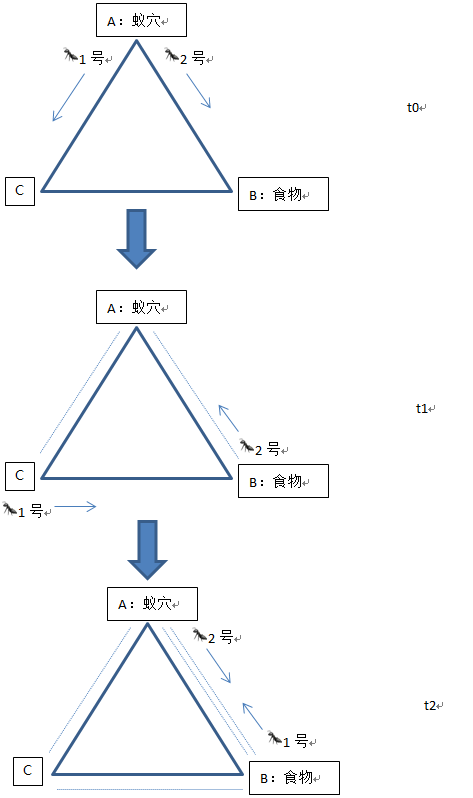以上便是蚁群算法的基本原理。
接下来介绍蚁群算法的数学模型，我们以TSP问题为例来进行说明。
TSP（Traveling Salesman Problem）问题，中文叫做旅行商问题：什么是TSP问题，也许有些人还不太清楚。这里做个简要的介绍。TSP问题及著名的旅行商问题，记得第一次接触这个问题是在本科时学习运筹学课程时碰到的一个问题，因此，这个问题是运筹学领域或也可以说是数学领域中的一个著名的问题。这个问题简单描述就是：假设一个旅行商人，他要遍历n个城市，但是每个城市只能遍历一次，最终还要回到最初所在的城市，要求制定一个遍历方案，使经过的总路程最短。
接下来便用蚁群算法对这个问题进行求解：
首先列出一些蚁群算法中涉及到的参数及其符号：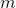：蚂蚁数量，约为城市数量的1.5倍。如果蚂蚁数量过大，则每条路径上的信息素浓度趋于平均，正反馈作用减弱，从而导致收敛速度减慢；如果过小，则可能导致一些从未搜索过的路径信息素浓度减小为0，导致过早收敛，解的全局最优性降低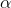：信息素因子，反映了蚂蚁运动过程中积累的信息量在指导蚁群搜索中的相对重要程度，取值范围通常在[1, 4]之间。如果信息素因子值设置过大，则容易使随机搜索性减弱；其值过小容易过早陷入局部最优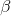：启发函数因子，反映了启发式信息在指导蚁群搜索中的相对重要程度，取值范围在[3, 4.5]之间。如果值设置过大，虽然收敛速度加快，但是易陷入局部最优；其值过小，蚁群易陷入纯粹的随机搜索，很难找到最优解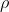：信息素挥发因子，反映了信息素的消失水平，相反的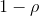反映了信息素的保持水平，取值范围通常在[0.2, 0.5]之间。当取值过大时，容易影响随机性和全局最优性；反之，收敛速度降低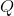：信息素常数，表示蚂蚁遍历一次所有城市所释放的信息素总量。越大则收敛速度越快，但是容易陷入局部最优；反之会影响收敛速度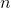：城市数量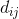：城市i到城市j之间的距离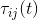：t时刻，城市i与城市j之间的信息素浓度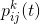：t时刻，蚂蚁k从城市i向城市j转移的概率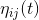：启发函数，表示蚂蚁从城市i转移到城市j的期望程度，这里我们取值为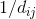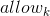：蚂蚁k待访城市的集合，初始时刻中有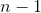个元素，即排除掉蚂蚁一开始所在城市以外的其他城市，随着时间推移，其中的城市越来越少，直到为空，表示遍历完所有的城市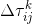：表示在所有蚂蚁遍历完所有城市时，第k只蚂蚁对城市i与城市j之间信息素浓度总增加量的贡献量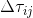：表示所有蚂蚁遍历完所有城市时，城市i与城市j之间信息素浓度的累积增加量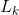：表示蚂蚁k遍历完所有城市后经历的总路程长度
接下来给出三个公式：
公式一：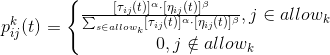从公式中可以看出信息素因子为信息素浓度的指数，启发函数因子为启发函数的指数，这样便很好理解这两个参数所起到的作用了，分别决定了信息素浓度以及转移期望对于蚂蚁k从城市i转移到城市j的可能性的贡献程度。
公式二：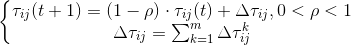这个公式反映了信息素浓度的迭代更新规律，可以看出，所有蚂蚁遍历完一次所有城市后，当前信息素浓度由两部分组成，第一部分即上次所有蚂蚁遍历完所有城市后路径上信息素的残留，第二部分为本次所有蚂蚁遍历完所有城市后每条路径上的信息素的新增量。
公式三：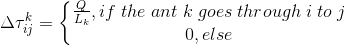公式三反映了每只蚂蚁对于自己经过的城市之间路径上信息素浓度的贡献量，可以看出，其经历的路程越长，则对于沿途上信息素浓度的贡献量也就越低，如果尽力的路程越短，则对于沿途上信息素浓度的贡献量也就越高，结合公式二可以看出，信息素浓度累积贡献量大的路径被选择的概率也就大，这就是为什么能够选出最短路径的原因。关于的计算还有一些其他模型，这里就不详细介绍了，我们这里给出的是ant cycle system模型，也是TSP问题中常用的一种模型。
最后给出蚁群算法解决TSP问题的流程：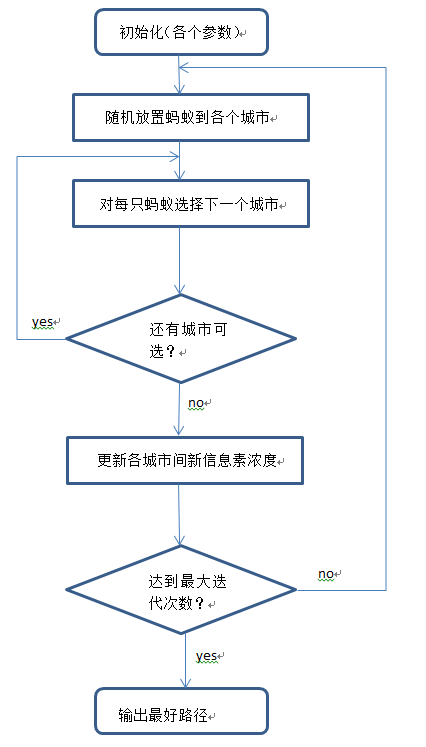组合参数设计策略：
由于蚁群算法对于参数的敏感程度较高，参数设置的好，算法的结果也就好，参数设置的不好则运行结果也就不好。
以上便是蚁群算法的基本原理，以下附上本人用python编写的关于蚁群算法的程序（程序中的参数需要根据具体问题自己进行调整）：
import os
import numpy as np
from numpy import random as rd
import matplotlib.pyplot as plt
np.set_printoptions(linewidth=1000, suppress=True)
# 在D盘根目录放置存放城市间距离矩阵的.txt文件distance.txt，或者存放城市坐标的.txt文件coordinate.txt，城市坐标排成一行，每个坐标用(x,y)表示。
###########distance.txt内容示例##################
"""
0 80 31 83 82 54 100 53
80 0 58 82 26 35 10 20
31 58 0 52 82 86 90 75
83 82 52 0 14 29 41 34
82 26 82 14 0 60 28 51
54 35 86 29 60 0 44 66
100 10 90 41 28 44 0 8
53 20 75 34 51 66 8 0
"""
# 如果为n行n列，则说明有n个城市，从第一行到最后一行分别代表城市0到城市n-1，第一列到最后一列也分别代表城市0到城市n-1
# 其中每个元素代表两个城市间的距离，对角线为0是因为自己到自己的距离为0
#############################################
# txt文件中同一行的每个数据之间用空格间隔
# 如果在D盘下两个文件均存在，则只读取distance.txt中的内容
# 根据具体问题复杂程度需要具体调整蚁群算法中的相关参数
os.chdir('D:')

class ACA(object):

def __init__(self, m, pher_0, alpha, beta, rho, Q, iter_times):
'''

:param Q: 信息素常数，表示每只蚂蚁循环一次释放的信息素总量，因此如果选择的路径长度长的话，则路径上信息素浓度Q/L就低
:param m: 蚂蚁数量，应该大约是城市数量的1.5被
:param pher_0: 每条路径上的初始时刻信息素浓度
:param alpha: 信息素因子
:param beta: 启发函数因子
:param rho: 信息素挥发程度，取值在0到1之间
:param iter_times: 算法的迭代次数（外层循环次数，所有蚂蚁遍历完一次所有城市为一次外层循环，所有蚂蚁每遍历一个城市为一次内层循环）
'''
self.iter_times = iter_times
self.Q = Q
self.rho = rho
self.alpha = alpha
self.beta = beta
self.m = m
# self.distance_mat为城市间距离矩阵
if os.path.exists("./distance.txt"):
elif os.path.exists("./coordinate.txt"):
self.city_coordinate = [eval(i) for i in np.loadtxt(fname="./coordinate.txt", dtype=np.object)]
print("城市坐标（0号城市~%d号城市）" % (len(self.city_coordinate) - 1), self.city_coordinate)
self.distance_mat = self.calc_distance(self.city_coordinate)
else:
raise Exception("请按照指定方式命名txt文件名，如果是城市间距离矩阵以distance.txt命名，如果是城市坐标则以coordinate.txt命名")
print("城市间距离矩阵：\n", self.distance_mat)
assert np.all(np.logical_not(np.array([self.distance_mat[i, i]
for i in range(self.distance_mat.shape)], dtype=np.bool))), "距离矩阵有误，对角线元素应该为0"
# self.pher_mat表示城市间信息素浓度矩阵，初始时刻各个城市间信息素浓度均为pher_0
self.pher_mat = np.array([[pher_0] * self.distance_mat.shape] * self.distance_mat.shape)
# 随机放置蚂蚁初始位
self.ant_init_position = rd.randint(0, self.distance_mat.shape, self.m)
# 启发函数
self.heu_f = 1 / (self.distance_mat + np.eye(self.distance_mat.shape, dtype=np.float64))
# self.best_lenth以及self.best_path用来存放每一次迭代最优路径长度以及最优路径
self.best_lenth = []
self.best_path = []

def run(self):
for i in range(self.iter_times):
# passed_city用来记录每只蚂蚁当前时刻已经路过的城市
passed_city = [[city] for city in self.ant_init_position]
for i in range(self.distance_mat.shape - 1):
# 一次内循环代表所有蚂蚁遍历了一个城市，排除自己初始化时所在城市，每只蚂蚁应该遍历self.distance_mat.shape - 1个城市，
# 因此内循环应该循环self.distance_mat.shape - 1次
for passed_ct in passed_city:
# 用循环变量passed_ct依次遍历每一只蚂蚁已经路过的城市, 每次遍历出的是一个列表
# allow_city为当前蚂蚁还没有遍历到的城市
allow_city = list(set(range(self.distance_mat.shape)) - set(passed_ct))
if len(allow_city) == 1:
passed_ct.extend(allow_city)
continue
# 每只遍历到的蚂蚁从其还没有遍历过的城市allow_city中选择下一个城市select_city,选择依据为概率p_i_j，
# 建立一个列表p，表示当前蚂蚁在当前城市到allow_city中每一个城市的概率
p = []
for allow_ct in allow_city:
p_dividend = self.pher_mat[passed_ct[-1], allow_ct] ** self.alpha * self.heu_f[passed_ct[-1], allow_ct] ** self.beta
p_divisor = 0
for allow_ct_inner in allow_city:
p_divisor += self.pher_mat[passed_ct[-1], allow_ct_inner] ** self.alpha * self.heu_f[passed_ct[-1], allow_ct_inner] ** self.beta
p_ = p_dividend / p_divisor
p.append(p_)
select_city_index = p.index(max(p))
# 将当前蚂蚁选择出的下一个遍历的城市select_city添加到当前蚂蚁已遍历的城市passed_ct中
select_city = allow_city[select_city_index]
passed_ct.append(select_city)
# 更新信息素浓度
delta_pher_k = []
delta_pher_mat = np.zeros(self.distance_mat.shape)
for ant_passed_city in passed_city:
accumulate_lenth = self.calc_passed_lenth(ant_passed_city)
delta_pher_k.append(self.Q / accumulate_lenth)
for i in range(self.m):
for k in range(self.distance_mat.shape - 1):
delta_pher_mat[passed_city[i][k], passed_city[i][k + 1]] += delta_pher_k[i]
delta_pher_mat[passed_city[i][-1], passed_city[i]] += delta_pher_k[i]
self.pher_mat = (1 - self.rho) * self.pher_mat + delta_pher_mat
# 所有蚂蚁遍历过一遍所有城市后再次初始化所有蚂蚁的所在城市
self.ant_init_position = rd.randint(0, self.distance_mat.shape, self.m)
ant_count_passed_path = []
for i in range(self.m):
ant_count_passed_path.append(passed_city.count(passed_city[i]))
best_path_index = ant_count_passed_path.index(max(ant_count_passed_path))
self.best_path.append(passed_city[best_path_index])
self.best_lenth.append(self.calc_passed_lenth(passed_city[best_path_index]))
print("最短路径为(城市编号)：", self.best_path[-1] + self.best_path[-1][:1])
print("最短路径长度为：", self.best_lenth[-1])

def calc_passed_lenth(self, passed_city):
lenth = 0
for i in range(len(passed_city) - 1):
lenth += self.distance_mat[passed_city[i], passed_city[i + 1]]
lenth += self.distance_mat[passed_city[-1], passed_city]
return lenth

def calc_distance(self, city_coordinate):
"""

:param city_coordinate_mat: 城市坐标
:return:
"""
distance = [np.sqrt(np.sum((np.array(i) - np.array(j)) ** 2)) for i in city_coordinate for j in city_coordinate]
distance = np.array(distance).reshape(len(city_coordinate), len(city_coordinate))
return distance

def draw(self):
fig = plt.figure()
ax = plt.subplot(1, 1, 1)
ax.plot(np.arange(self.iter_times), self.best_lenth, "*-", color="r", label="best lenth of path every iteration")
ax.set_xlabel("iteration times")
ax.set_ylabel("lenth")
ax.legend()
plt.show()
try:
len(self.city_coordinate)
except Exception as e:
pass
else:
fig = plt.figure()
ax = plt.subplot(1, 1, 1)
x = [self.city_coordinate[i] for i in self.best_path[-1] + self.best_path[-1][:1]]
y = [self.city_coordinate[i] for i in self.best_path[-1] + self.best_path[-1][:1]]
ax.plot(x, y, "o--", color="r", label="route", linewidth=2.5)
count = 0
for x_, y_ in zip(x, y):
count += 1
if count == len(x):
break
plt.text(x_, y_, "%d(city_%d)" % (count, self.best_path[-1][count - 1]), fontsize=10)
ax.set_xlabel("x")
ax.set_ylabel("y")
ax.set_title("The order of traversing the city")
ax.legend()
ax.grid()
plt.show()

def main():
aca = ACA(12, 6, 4.5, 3, 0.6, 500, 2000)
aca.run()
aca.draw()

if __name__ == "__main__":
main()


展开全文• 该压缩包是关于车辆路径问题的求解算法，蚁群算法具有收敛性强的特点
• 根据10个城市和30个城市的数据，用蚁群算法选择出来最短的路径
• Matlab中蚁群算法求解连续函数优化的原程序-蚁群算法连续函数优化问题matlab程序.rar 蚁群算法求解连续函数优化的原程序 所含文件： Figure41.jpg 蚁群算法连续函数优化问题matlab程序matlab...